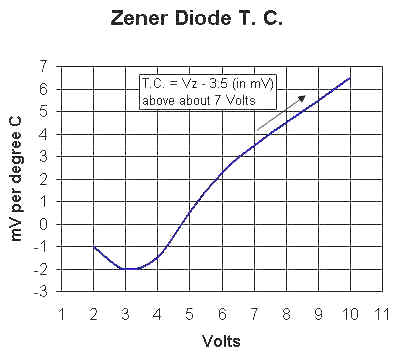The formula for zener diode temperature coefficients above 7 volts uses volts to calculate millivolts. For example, a 50 volt zener will have about 50 - 3.5 = 46.5 mV/degree temp-co. In other words, divide the result by 1000.

Notes:

• A 3.6 volt zener exhibits about -2mV/degree which can come in handy for biasing a transistor circuit as can a 5.6 volt zener with its +2mV/degree coefficient.

• The temperature coefficients shown are approximate and will vary with diode type and current.

• Temperature compensated diodes are available that use silicon diodes in series with a zener diode to achieve a very low temperature coefficient at a fixed, specified current. For example, a 1N821 is a 6.2 volt compensated zener made with an ordinary silicon diode in series with a 5.6 volt zener. At 7.5mA bias, the zener's positive temp-co cancels the silicon diode's -2mV/degree temp-co.